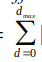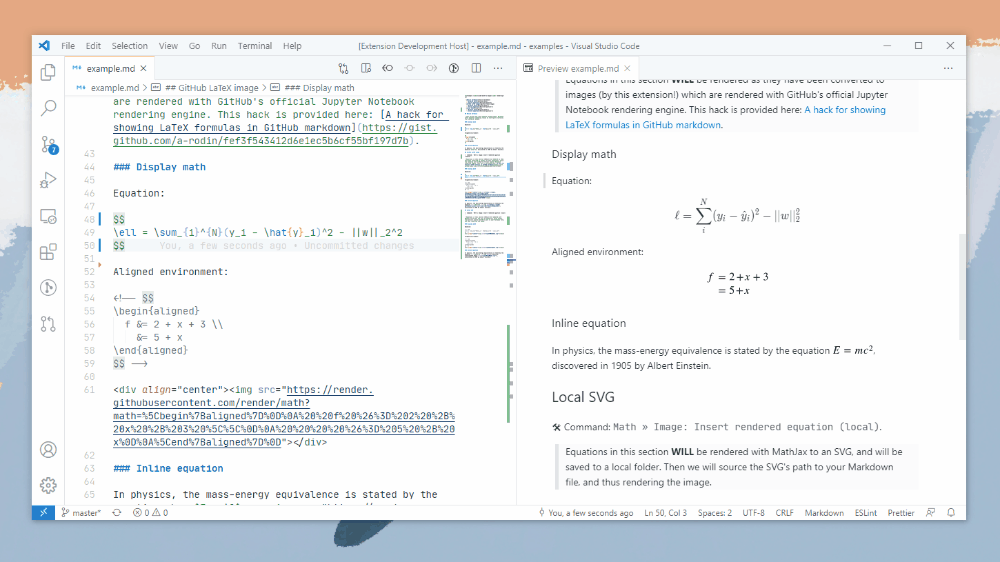{{ message }}

Instantly share code, notes, and snippets.

# a-rodin/A hack for showing LaTeX formulas in GitHub markdown.md

Last active Aug 6, 2022

## Problem

A lot of GitHub projects need to have pretty math formulas in READMEs, wikis or other markdown pages. The desired approach would be to just write inline LaTeX-style formulas like this:

$e^{i \pi} = -1$

Unfortunately, GitHub does not support inline formulas. The issue is tracked here.

## Investigation

However, it does support them in Jupyter notebooks, scroll below to see an example. One might question how does it work in notebooks. It turns out that instead of relying on MathJax as nbviewer does, GitHub's notebooks renderer converts inline math to images with source URLs that look like this:

https://render.githubusercontent.com/render/math?math=e%5E%7Bi%20%5Cpi%7D%20%3D%20-1&mode=inline

Inspecting the URL, it is possible to notice that:

1. The &mode=inline part is not required, the URL still returns the same image without it:

2. Modern browsers encode URLs automatically, thus this link would work as well:

## Solution

So the solution would be to insert this code in Markdown files:

<img src="https://render.githubusercontent.com/render/math?math=e^{i \pi} = -1">

and such an image would be rendered as.

This is more clumsy than just $e^{i \pi} = -1$, but it is still possible to write the formula by hand directly to the Markdown file this way.

Note that this syntax for image insertion would not work because the URL contains spaces:

![formula](https://render.githubusercontent.com/render/math?math=e^{i \pi} = -1)

I made a small app that allows to generate Markdown code from LaTeX using the method described above automatically.

Sorry, we cannot display this file.
Sorry, this file is invalid so it cannot be displayed.

### AdiPratapSingh commented Feb 17, 2022

Excellent work. Could you make it available for the dark background mode too?

Thanks

### npanuhin commented Feb 21, 2022 • edited

Solved the color issue using theme context for images. White color for dark mode and black color for light mode at the same time:

<img src="https://render.githubusercontent.com/render/math?math={\L = -\sum_{j}[T_{j}ln(O_{j})] + \frac{\lambda W_{ij}^{2}}{2} \rightarrow \text{one-hot} \rightarrow -ln(O_{c}) + \frac{\lambda W_{ij}^{2}}{2}}#gh-light-mode-only">
<img src="https://render.githubusercontent.com/render/math?math={\color{white}\L = -\sum_{j}[T_{j}ln(O_{j})] + \frac{\lambda W_{ij}^{2}}{2} \rightarrow \text{one-hot} \rightarrow -ln(O_{c}) + \frac{\lambda W_{ij}^{2}}{2}}#gh-dark-mode-only">

### gobalkrishnan-engineer commented Feb 22, 2022

How to view the \chemfig in the overleaf latex

### gobalkrishnan-engineer commented Feb 22, 2022

https://www.overleaf.com/learn/latex/Chemistry_formulae and other DNA, RNA, medicial, electronic, etc. latex in

### JulianChia commented Mar 12, 2022

How do I showusing <img src="https://render.githubusercontent.com/render/math?{}> ?

### RicardoLera commented Mar 13, 2022

@JulianChia You can use:
<img src="https://render.githubusercontent.com/render/math?math={\sum_{d=0}^{d_{max}}}">
You can edit more complex equations at https://latex.codecogs.com/eqneditor/editor.php, for example.

### JulianChia commented Mar 15, 2022 • edited

@JulianChia You can use: <img src="https://render.githubusercontent.com/render/math?math={\sum_{d=0}^{d_{max}}}"> You can edit more complex equations at https://latex.codecogs.com/eqneditor/editor.php, for example.

Thanks for the link. I also discovered your answer is incomplete. Instead, it should be <img src="https://render.githubusercontent.com/render/math?math={\displaystyle\sum_{d=0}^{d_{max}}}">

### HarvsG commented Apr 1, 2022 • edited

<img src="https://render.githubusercontent.com/render/math?math={P(A|B)=\frac{\P(B|A)\P(A)}{\P(B|A)\P(A)\%2BP(B|\neg A)\P(\neg A)}}##gh-light-mode-only">
<img src="https://render.githubusercontent.com/render/math?math={\color{white}\P(A|B)=\frac{\P(B|A)\P(A)}{\P(B|A)\P(A)\%2BP(B|\neg A)\P(\neg A)}}#gh-dark-mode-only">

### kozross commented Apr 4, 2022

@HarvsG: I tried your solution in a Github wiki page, and I instead get both versions, rather than the one corresponding to my theme.

### npanuhin commented Apr 5, 2022

Indeed, this approach does not work for GitHub wiki - there are no style rules for #gh-light/dark-mode-only

### chr-wei commented Apr 7, 2022

Great solution (at least for .md files)! I wish this was natively integrated into GitHub .md files.

### howardchina commented Apr 25, 2022

Hi guys, me and my friends made a VS Code extension to convert math equations into rendered SVGs here: GitHub- vscode-math-to-image / VS Marketplace - Math to Image. We used the method mentioned in this gist to render external SVGs with GitHub math rendering servers. We also added a feature to render them into local SVGs with MathJax. Feel free to try it out!Just downloaded it. It seems to be broken for the remote option.

nice solution

### npanuhin commented May 15, 2022 • edited

For some reasons the "+" sings disappear with the https://render.githubusercontent.com/render/math under a sqrt operator

The + character has a special meaning in a URL => it means whitespace -  . If you want to use the literal + sign, you need to URL encode it to %2b. (source, one more example)### barrettj12 commented May 20, 2022

Looks like LaTeX is supported in GitHub Markdown now:
$$\sum_{n=0} \frac{1}{n!}\ =\ e$$

### RicardoLera commented May 20, 2022 • edited

https://github.blog/2022-05-19-math-support-in-markdown/
Yep, that's true. So much for all the hours I spent making this method work.

Although considering this is the first place Google takes you when looking this problem up, maybe we managed to show the developers that this is an important problem to take care of.

### bgraham89 commented May 20, 2022

It's working to some extent which is awesome, but there are cases where doesn't. I'm not sure if that's caused by mathjax rendering or not. Examples include :

• addition $+$,
• subtraction $-$,
• multiplication $\times$,
• division $\div$.

and set brackets:

$$\mathbb{N} = { 1, 2, 3, ... }$$

### npanuhin commented May 20, 2022 • edited

I'm glad GitHub finally decided to introduce MathJax. However:

UPD: LaTeX kerning is fixed (for) now

I find it weird that there is no indentation. For example ($(1 ⩽ n ⩽ 100\ 000)$):
$(1 ⩽ n ⩽ 100\ 000)$ or $O(n \log n)$ or $(a_i ∈ {0, 1})$
To make these equations look normal, I have to manually specify that spaces should be preserved ($(1\ ⩽\ n\ ⩽\ 100\ 000)$):
$(1\ ⩽\ n\ ⩽\ 100\ 000)$ and $O(n\ \log\ n)$ and $(a_i\ ∈\ {0,\ 1})$

UPD: As of now LaTeX works in lists and tables. Headers support is still pending

1. $And\ of\ course\ it\ completely\ doesn't\ work\ in\ lists...$

### eo1989 commented May 22, 2022 • edited

testing testing 1.. 2.. 3..

$a^2$ $+$ $b^2$ $=$ $c^2$

### npanuhin commented May 22, 2022 • edited

UPD: LaTeX kerning is fixed (for) now

Seems like you found another way to solve the kerning problem
$a^2 + b^2 = c^2$ ($a^2 + b^2 = c^2$)
$a^2\ +\ b^2\ =\ c^2$ ($a^2\ +\ b^2\ =\ c^2$)
$a^2$ $+$ $b^2$ $=$ $c^2$ ($a^2$ $+$ $b^2$ $=$ $c^2$)

### RussellDash332 commented May 23, 2022

Cool stuff
$$a^2+b^2=c^2$$

$$\int_0^1f(x)dx=\int_{-1}^0f(-x)dx$$

### nschloe commented May 23, 2022

Somebody pointed it out earlier, but here's my blog post that explains in detail what's wrong with math support. It covers everything that's been reported here.

tldr:

• GitHub first applies the Markdown parser, then the math parser
• The markdown parser messes with the math (because it thinks $ and $\$ is just text). For example it removes backslashes in @bgraham89's example.
• If there's Markdown/HTML syntax in math (e.g., a <b > c), it won't render
• If there's math in Markdown syntax (e.g., in lists and headers), it won't render
• Kerning and sizing is probably a MathJax config problem, hopefully easily fixable

Yay :)

### HinnyTsang commented Jul 11, 2022

Now github supports it : https://github.blog/2022-05-19-math-support-in-markdown/

But it is still not supported on GitHub Wiki

### HinnyTsang commented Jul 11, 2022

@HarvsG: I tried your solution in a Github wiki page, and I instead get both versions, rather than the one corresponding to my theme.

This approach seems to be working in the github wiki. But it seems like only working with google chart.